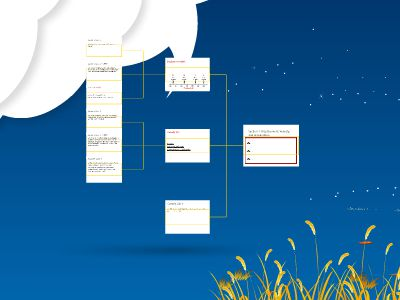Publications: 82 | Followers: 0

## Section 1_ Displacement, Velocity, and Acceleration

Publish on Category: Birds 0

Section 1: Displacement, Velocity, and Acceleration
Displacement (ΔX):__________
Velocity (V) :_____________________
Speed:Average Velocity:Instantaneous Velocity:
Q1:What is the average velocity of a car that moved 60km in 3 hours?
Sample Calc 1:
Q2:How far will a car travel in 25 min at12m/s?
Sample Calc 2:
Q3: Car A is traveling 30m/s at mile marker 50 meters and car B is traveling 70m/s at mile marker zero. Find the mile marker where the will meet.
Sample Calc 3: TUFFY
Acceleration (a) :________________
Acceleration Equation:Displacement with Acceleration:
Q1:If a car accelerates from 3 m/s to 12 m/s in 3 seconds, what is the car's average acceleration?Q2: Find Howfarittraveled:
Sample Calc 1:
Q3: Car A starts at miles marker zero and begins to accelerate forward at a rate of 10m/s2. Car B is heading in the opposite direction at mile marker 400 meters and begins to accelerate at -20m/s. Find where they meet.
Sample Calc 2: TUFFY
Q: You started to run at 10m/swhen you left your house and you arrived at school300 secondslater. Assuming that your average acceleration was 30m/s2,how fast were you running when you arrived?
Sample Calc 3

0

Embed

Share